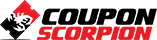# Fundamentals Of Fluid Mechanics

Fluid Statics, Forces on Plane and Curved Surfaces, Manometers, Bernoulli Equation, Linear Momentum, Mass Conservation

### What you’ll learn

• Determine the variation of pressure in a fluid at rest
• Calculate the forces and moments exerted by a fluid at rest on plane or curved submerged surfaces
• Have a working knowledge of viscosity and the consequences of the frictional effects it causes in fluid flow
• Calculate the capillary rise (or drop) in tubes due to the surface tension effect
• Utilize Integral relations for a control volume and differential relations for fluid particles to analyze fluid flow problems.
• Use control volume analysis to determine the forces associated with fluid flow

### Requirements

• Physics and Calculus

### Description

Introduction to fluid properties, viscosity, surface tension, pressure distribution in fluid, hydrostatic forces on plane and curved surfaces, buoyancy , and pressure measurement using manometers and barometers. Integral relations for a control volume, mass conservation, linear momentum equation, energy and Bernoulli equations are discussed.

37 problems are solved in order to bridge the gap between knowledge and application.

Author(s): Prof. Samer

Deal Score+5
\$12.99 \$94.99